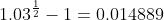Scan QR code or get instant email to install app

Question:

# An investor is considering the purchase of Security X, which matures in ten years and has a par value of SI,000. During the first five years, X has a 6% coupon with quarterly payments. During the remaining five years, X has an 8% coupon with quarterly payments. The face value is paid at maturity. A second 10-year security, Security Z, has a 6% semiannual coupon and is selling at par. Assuming that X has the same bond equivalent yield as Z, the price of Security X is closest to:

A \$1,067.
explanation

1212The bond equivalent yield rate on the par bond (Z) is 6% or a 3% semiannual rate. The equivalent quarterly rate,. Security X makes 20 quarterly payments of 15 and 20 quarterly payments of 20. We need to use the cash flow function as follows: CFQ - 0; CFj = 15; Fj = 20; CF9 = 20; CF3 = 1,020;
F3 = 1; I = 1.4889; CPT -> NPV = 1,067.27. Note that CF3 contains the final quarterly payment of 20 along with the 1,000 face value payment.